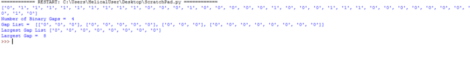# Binary Gaps with Python

#### Posted on August 16, 2019 by By admin, in Data Visualization | 0

Recently I saw a question on-line to find the Binary Gaps in a Binary Number.

#### What is a Binary Gap?

A binary gap within a positive integer N is any maximal sequence of consecutive zeros that is surrounded by ones at both ends in the binary representation of N.

Example:

Assuming you have Binary Number of 10001000001, the binary Gaps here are 2 because we have 10001 and 1000001 which interprets as gaps between Ones (1)

so if you are looking to expand your knowledge in python, this a good question once you have a good beginner knowledge in Python to solve.

Below is my solution:

```a  = '011111111100010000010001110000000010'
b  = list(str(a))
print(b)

notrue = []
largestbgaplist=[]
gaplist=[]
tfn = b.index('1')
#print("Index of the first one ",str(tfn))
if(tfn>0):
b = b[tfn:]
else:
b = b
#print(b)
tn = 0

for i in range(len(b)):
#print(b[i])
z = b[tn]
#print("Z = "+str(z)+" b[i] = "+b[i])
if(z == b[i] and tn!=i and b[i]==b[i-1]):
tn = i
if(z == b[i] and tn!=i and b[i]!=b[i-1]):
notrue.append(True)
#print("Old Index =", str(tn))
#print("New Index =", str(i))
xxx = b[tn+1:i]
tn = i
#print(xxx)
largestbgaplist.append(xxx)
#print("New Index Search = ", str(tn))
#print("i = ",str(i))

print("Number of Binary Gaps = ",str(len(notrue)))
print("Gap List = ",largestbgaplist)
print("Largest Gap List", max(largestbgaplist,default='Empty'))
largestgaplist = []
for items in largestbgaplist:
largestgap = items.count('0')
largestgaplist.append(largestgap)
print("Largest Gap = ",max(largestgaplist))
```

I kept in mind few Test Cases like:

1. if the Binary Number starts with 0
2. Ends with 0
3. Multiple 1’s

and also could find the largest Binary Gap in the Binary Number.

Example: 011111111100010000010001110000000010Feel free to test the code and comment your test cases.

In case if you have any queries please get us at support@helicaltech.com

Thank You
Sohail Izebhijie
BI Developer
Helical IT Solutions Pvt LtdBest Open Source Business Intelligence Software Helical Insight HereBest Open Source Business Intelligence Software Helical Insight is Here